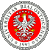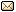XXXIX Workshop on Geometric Methods in Physics 27.06-3.07.2021 IX School on Geometry and Physics 21-25.06.2021
 Home First Announcement Travel and local information Lecturers (WGMP) Lecturers (SGP) Registration Photos Proceedings Previous Workshops Email

# Janusz Grabowski

## $\mathbb{Z}_2^n$-supermanifolds

The concept of a $\mathbb{Z}_2^n$-supermanifold will be presented with its local model made of formal power series with $\mathbb{Z}_2^n$-gradation and $\mathbb{Z}_2^n$-commutation rules. The classical Batchelor-Gawedzki theorem says that any smooth supermanifold is (non-canonocally) diffeomorphic to the superization' $\Pi E$ of a vector bundle $E$. It is also known that this result fails in the complex analytic category. We show that any smooth $\mathbb{Z}_2^n$-supermanifold is (non-canonocally) diffeomorphic to the superization' $\Pi E$ of an $n$-fold vector bundle $E$. The latter can be chosen split.

 Event sponsored by: University of BialystokWebpage by: Tomasz Golinski